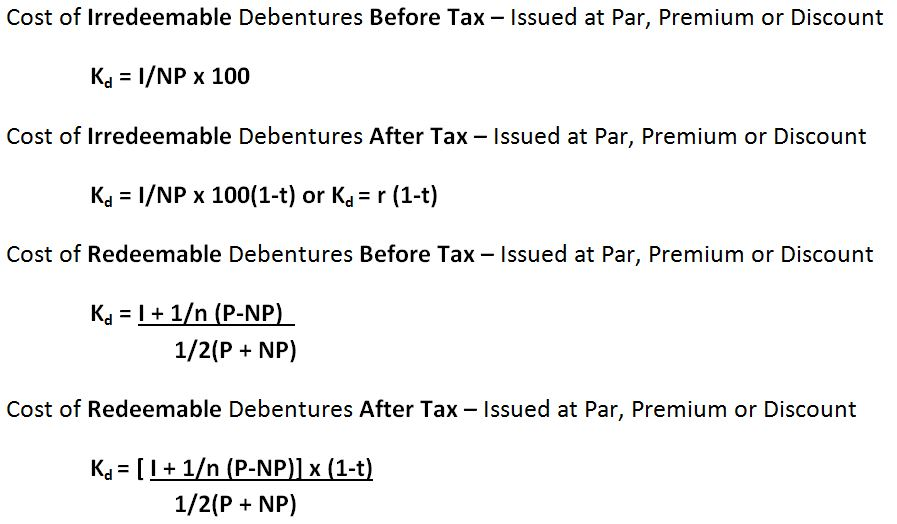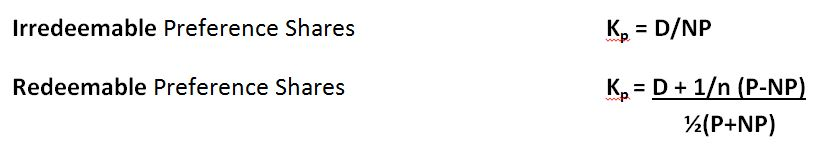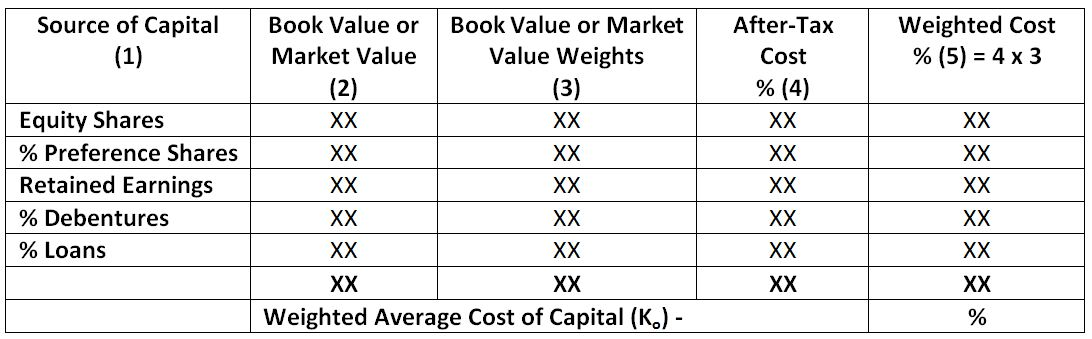# Cost of Capital – Introduction & Computation

The Cost of Capital is the minimum rate of return, that a company requires from its investments in order to ensure that the market value of its shares either increase or remain at the same level.

It simply refers to the minimum profit a firm requires from its investment in order to increase its market valve.

According to James Van Horne, Cost of Capital is “A cut-off rate for the allocation of capital to investments of project. It is the rate of return on a project that will leave unchanged the market price of stock”

## Assumptions/Characteristics of Cost of Capital

• Cost of capital is the indication of expectations of shareholders regarding returns from their investments and may not necessarily be in form of cash cost every time.
• It is the minimum rate of return required from investments to maintain market value of a firm`s equity shares.
• Computation of cost of capital requires consideration of the number and degree of risks associated with the project/business and is directly proportional to it i.e. cost of capital is high if the risks associated with the project/business are high and vice versa.

Cost of capital (Ke) = Risk free rate (Rf) + Risk Premium (Rp)

## Importance of Cost of Capital

• It is essential to compute cost of capital in order to determine an optimal capital structure of the business concern and take good Capital Budgeting Decisions.
• Cost of capital affects the capital structure and capital budgeting decisions which in turn affect the value of the firm. Therefore it also helps to evaluate the financial performance of a business
• It helps to evaluate various sources of finance and select the most profitable one.
• It helps to formulate an optimum mix of debt and equity capital.
• It helps to take major financial decisions such as market value of share, working capital requirements, earning capacity of securities, distribution of dividends etc.

## Computation of Cost of Capital

Computation of cost of capital consists of two important parts:

(I) Measurement of specific costs

(II) Measurement of overall cost of capital or WACC

### (I) Measurement of Specific Cost of Capital

It refers to the computation of cost related to each specific source of finance like:

• Cost of equity capital (Ke)
• Cost of debt/debenture capital (Kd)
• Cost of preference share capital (Kp)
• Cost of retained earnings (Kr)

Valuation of Cost of Equity (Ke) – It is the minimum rate of return required from equity financing investments to ensure growth of market value of a company. The various methods of computing cost of equity are as under –

 Dividend Yield Method Ke = D/NP x 100 OR D/MP x 100 Dividend Yield + Growth Ke = (D/NP) x 100 + g Earning Yield Method Ke = E/NP x 100 OR EPS/MP x 100 Earning Growth Method Ke = (E/NP) x 100 + g OR EPS/MP x 100 Realised Yield Method Ke = (W1 x W2 x W3…….Wn)1/n – 1 Wt = Dt + Pt          Pt-1 Capital Asset Pricing Model (CAPM) Ke = Rf + b (Km – Rf)

Here,

NP = Net Proceeds per share = Face value + Premium – Discount – Cost of Issue (if any)

D = Expected dividend per share

MP = Market Price per Share

g = Growth rate of dividends/earnings

E/EPS – Earnings per share

Wt = Wealth for the year t

Dt = Dividend per share at the end of year t

Pt = Price per share at the end of year 1

Pt-1 = Price per share at the beginning of the year

Rf = Risk Free Return Km = Market Return b = beta coefficient

Valuation of Cost of Debt (Kd) – Cost of Debt/Debentures refers to the rate of interest payable by a company on its issued debt instruments. Debentures issues by a company may be Redeemable or Irredeemable and may be computed before or after tax has been deducted. They may be issues at par, at premium or at a discount.Here,

NP = Net proceeds

When Shares are issued:

At Par – NP = Face value – Cost of Issue

At Premium – NP = Face value + Premium – Cost of Issue

At Discount – NP = Face value – Discount – Cost of Issue

I = Fixed Annual Interest Payable                   t = Tax rate

r = Interest Rate Payable                                 n = Number of Years

P = Par/Face/Redeemable Value of debentures

Valuation of Cost of Preference Shares (Kp) – It refers to the cost associated with the payment of dividend to preference shareholders according to their expectations. It can be simply defined as the minimum rate of return expected out investments made my preference shareholders in order to maintain and attract funds. Preference shares may be redeemable or Irredeemable.Here,

D = Annual Preference Dividend            N = Number of Years

P = Par/Face/Redeemable Value of Preference shares

Valuation of Cost of Retained Earnings (Kr) – Generally the cost of retained earnings is equal to the cost of equity share capital as both sources of funds belong to the owners of the company and they expect the same rate of return out of investments made from each source.

Cost of Retained Earnings may be simply defined as opportunity cost of additional dividends that shareholders would have received, if the retained earnings were distributed as dividends and not retained.

Kr = (D/NP + g) x (1-t) x (1-b)                    OR

Kr = Ke x (1-t) x (1-b)

Here,

D = Expected dividend                g = Growth Rate

NP = Net Proceeds of Equity shares  t = Tax rate

b = Cost of purchasing securities or brokerage cost  Ke = Cost of Equity capital

## (II) Measurement of Overall Cost of Capital or Weighted Average cost of capital (WACC)

Weighted average cost of capital is the weighted average cost of equity and debt capital, where weights are the amount of capital raised from each source.

The computation of the overall cost of capital (Ko) involves the following steps.

(a) Assigning weights to specific costs

(b) Multiplying the cost of each of the sources by the appropriate weights

(c) Dividing the total weighted cost by the total weights.

(d) The overall cost of capital is calculated with the following formula –

WACC or Ko = (Kd x Wd) + (Kp x Wp) + (Ke x We) + (Kr x Wr)

Here,

Ko = Overall cost of capital                        Kd = Cost of debt

Kp = Cost of preference share                  Ke = Cost of equity

Kr = Cost of retained earnings

Wd= Percentage of debt in the total capital

Wp = Percentage of preference share of the total capital

We = Percentage of equity shares of the total capital

Wr = Percentage of retained earnings of the total capital

### Format for Calculation of WACCNote: The term ‘Opportunity cost’, ‘Minimum required rate of return’, ‘Cost of capital’, ‘Discount rate’ and ‘Interest rate’ are all synonymous in financial management.